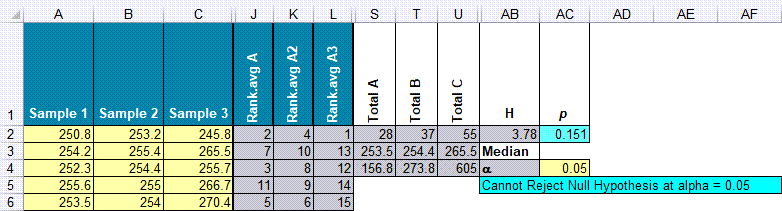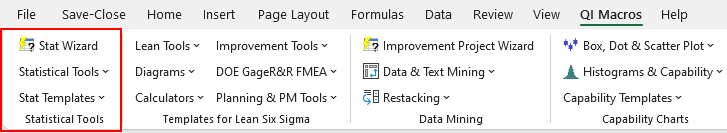# Want to Run a Kruskal-Wallis Test in Excel?#### When Should You Use the Kruskal-Wallis Test?

A Kruskal-Wallis test is like an Analysis of Variance (ANOVA). It is a non-parametric test that compares unmatched groups. Samples must be random, independent and five or more per sample.

Note: Excel does not do statistical tests of non-normal (i.e., not "bell shaped") data. QI Macros, adds this functionality with a set of of non-parametric statistical test templates.

### Kruskal-Wallis Example Using QI Macros Template

A golfer wants to compare three drivers to determine which one he can hit the longest. He hits five drives with each driver and measures the distance.

To conduct a Kruskal-Wallis test using QI Macros template:

1. Click on the QI Macros menu > Stat Templates > Kruskal-Wallis to open the non-parametric test template. Then select the "Kruskal-Wallis" tab.
2. Input your data into the yellow shaded cells in columns A:I.
3. QI Macros will perform the calculations in columns J:R and display the results in columns S:AA. In the example, below we have hidden the unused columns. (Note never delete rows or columns in QI Macros templates as you may accidentally break formulas.)
4.### Interpreting Kruskal-Wallis Results

Since p (0.151) is greater than alpha* (0.05), we cannot reject the null hypothesis that the distance of all three drivers is the same. In laymans terms, that means you would "accept the null hypothesis" = the distance is the same.

If p was less than alpha, we would reject the null hypothesis.

*Default Significance Level is 0.05*

H: This is the test statistic that helps you determine whether the medians of two (or more) groups are different, in combination with your p value.

#### Haven't you waited long enough? Start running Kruskal-Wallis tests in just minutes.